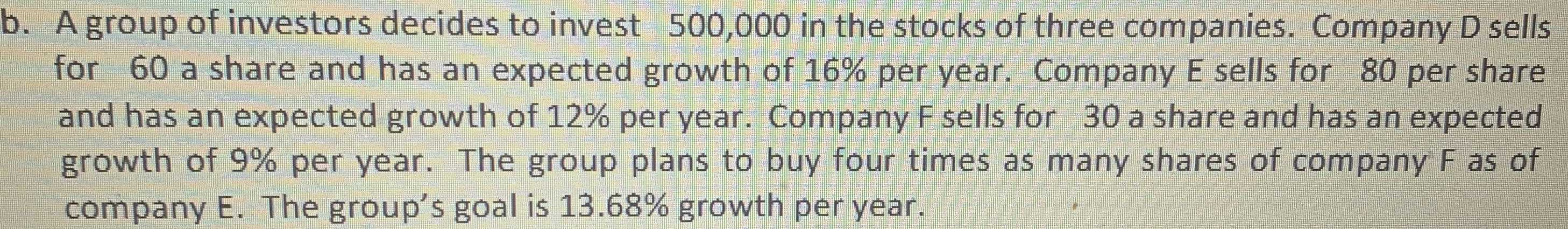### Still have math questions?A group of investors decides to invest $$500,000$$ in the stocks of three companies. Company D sells for $$60$$ a share and has an expected growth of $$16 \%$$ per year. Company E sells for $$80$$ per share and has an expected growth of $$12 \%$$ per year. Company F sells for $$30$$ a share and has an expected growth of $$9 \%$$ per year. The group plans to buy four times as many shares of company F as of company E. The group's goal is $$13.68 \%$$ growth per year.
$$x= 5000\\y= 1000\\4y= 4000$$